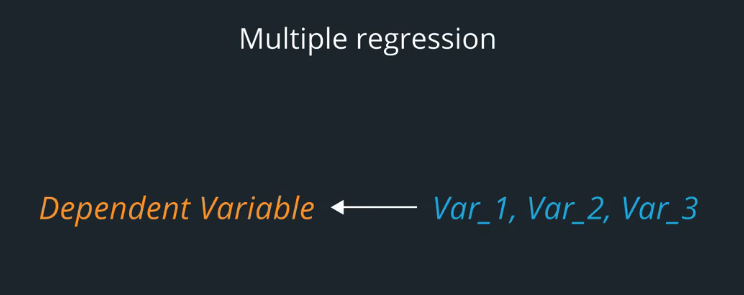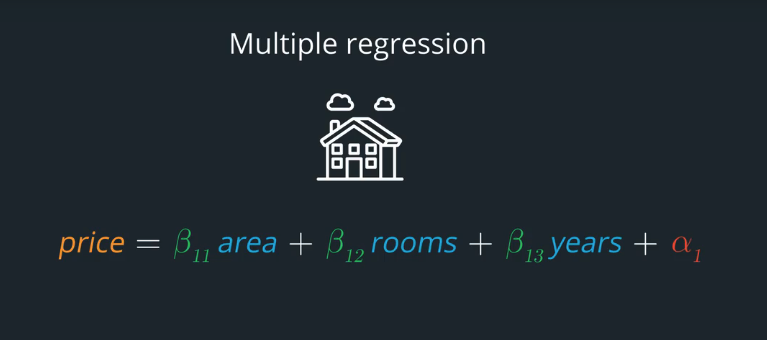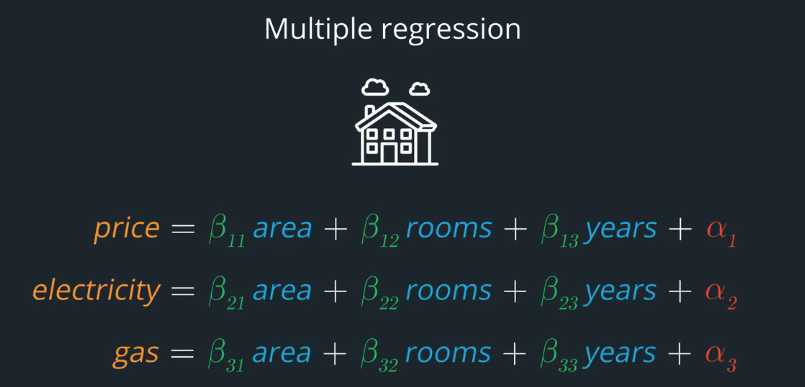# AI For Trading: Multivariate Linear Regression (20)Earlier,we touched on using multiple independent variables to predict a single dependent varialble. We call this multiple regression.

In this example,we are using a house's area, number of rooms and number of years since the room was built. All three are variables that we use to predict the house price .We can go one step further and choose more than one dependent variable that we wish to predict .Now,we're not just predicting the house price, but also trying to predict the home's electricity consumption and gas consumption. When we tried to predict more than one dependent variable at the same time .
this is called multivariate regression.### Multivariate multiple regression

When we're using more than one independent variable to predict more than one dependent variable, this is called multiple regression. This appears rather complicated,but bebing familiar with multivariate regression will help you later when you learn about time series and pairs trading.